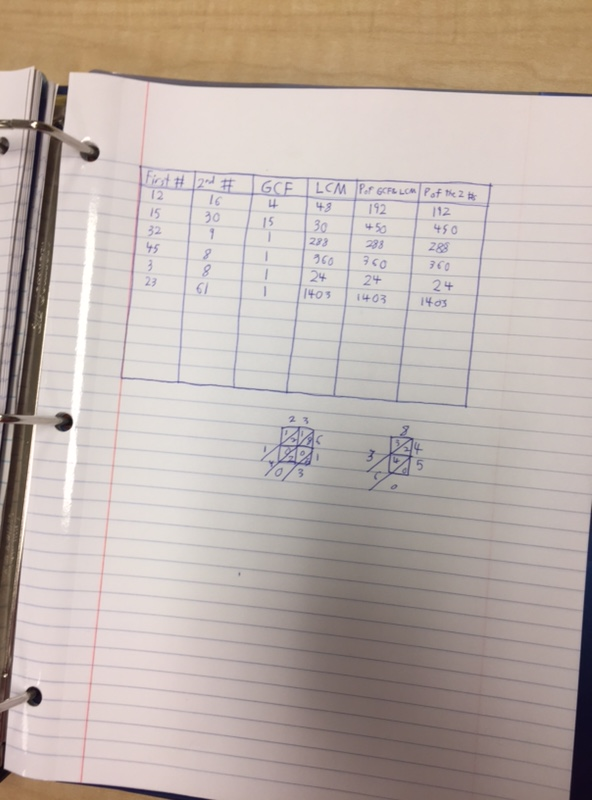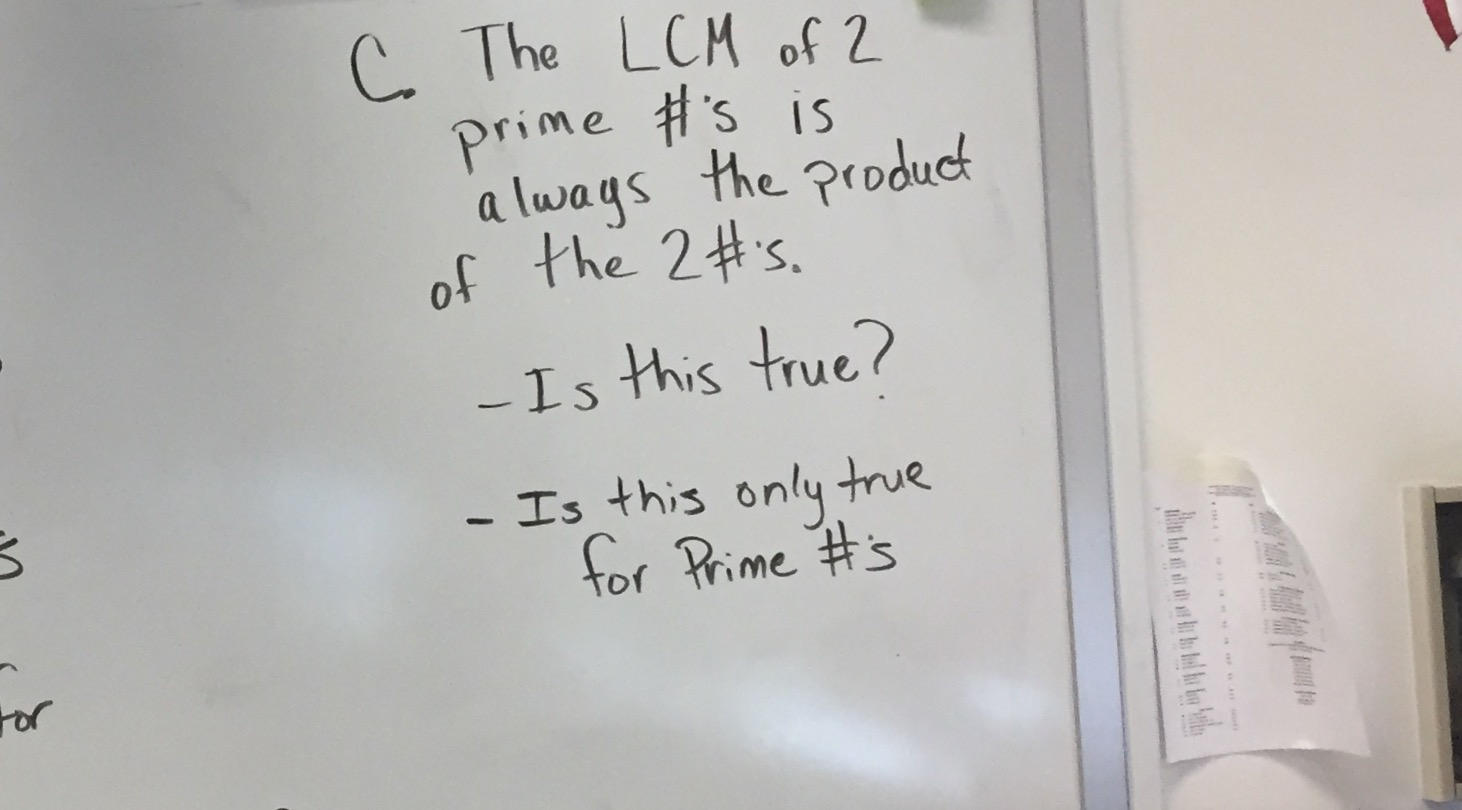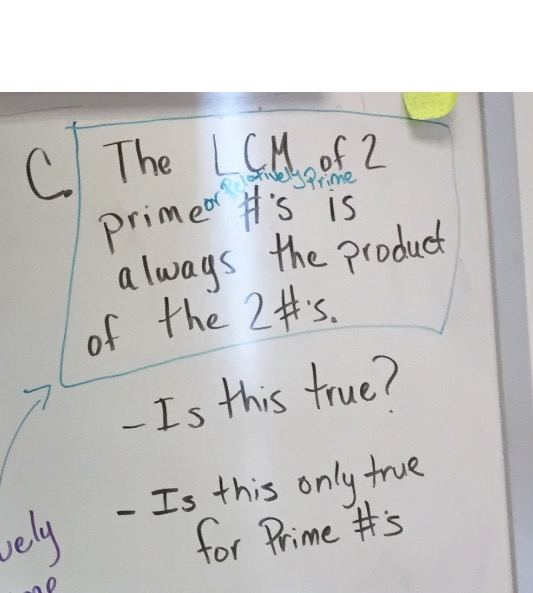# Gr6 Math Week of 11/13/17

In the last week, we focused on contextual situations in which determining Least Common Multiple (LCM) and Greatest Common Factor (GCF) are helpful in determining a solution.

This is a situation that occurs in real life all the time. For example, if my friend and I have a habit of attending a restaurant regularly, and I go every 4 days, but my friend  goes every 5 days. We can use LCM to determine in many days can we expect to see each other again?

We explored this concept in several situations. A few examples are:

1: A large Ferris wheel makes one revolution in 60 seconds. A small Ferris wheel makes one revolution in 20 seconds, how many seconds pass before the riders who start at the same time are both at the bottom again?

2: Suppose 13-year and 17 year cicadas both appear this year. When will both types of cicadas next appear together?

3. Mr. M and his 23 students are planning to have hot dogs at their class picinc. Hot dogs come in packages of 12 and buns come in pacakages of 8. What is the least number of packages of hot dogs and the least number of packages of buns he can buy so that there are no leftovers?

In doing this work, students made some ‘conjectures’ about the relationships between the numbers when looking for GCF and LCM. In math, the term “conjecture” refers to a specific statement that is thought to be true but has not been proven. Today, we explored these conjectures and revised our thinking so that we could have a clear idea of the important relationships of factors and multiples when the numbers you are looking at have certain characteristics.

To do this, we completed a table, and used the information contained in it, using given number pairs and pairs we tried on our own, to help turn our conjectures into things we know for sure about the relationships of a set of numbers.Student notebook as he completed the table and looked at relationships

Conjecture A:To look more closely at this, we looked at the relationship between 15 and 30, and other examples like 2 and 4 or 15 and 45, and determined that if the smaller of a set of 2 numbers is a factor of the larger number, then the LCM will be the larger number, and the GCF will always be the smaller number.

Then, we looked at the next conjecture:When examining this relationship that we noticed in the Cicada problem, students saw that the GCF of 2 prime numbers would always be one, and realized this was not just when you are dealing with 13 year and 17 year Cicadas. They decided that this made sense because if 2 numbers are prime the only factors they have are one and themselves, therefore there would be no overlap in factors other than 1. HOWEVER, they also noticed that there were other number pairs where one number was Prime and the other was not, such as 3 and 8, or where both numbers were composite, such as 45 and 8, but the GCF was still 1. This was explained by the concept of 2 numbers being Relatively Prime. Relatively Prime numbers are a pair of numbers where the only common factor is 1.

Using this new information about Relatively Prime numbers, we looked at our 3rd conjecture:And revised it to say:The LCM of 2 Prime or Relatively Prime number is always the product of the 2 numbers.

:

In both 601 and 602, students were able to see the relationships and gave clear examples of why the conjectures were true relationships, or revise the wording to reflect how the patterns worked from their charts.

In the coming days we will look closely at Prime Factorization as we think more about the characteristics of numbers such as factors and multiples to answer questions.